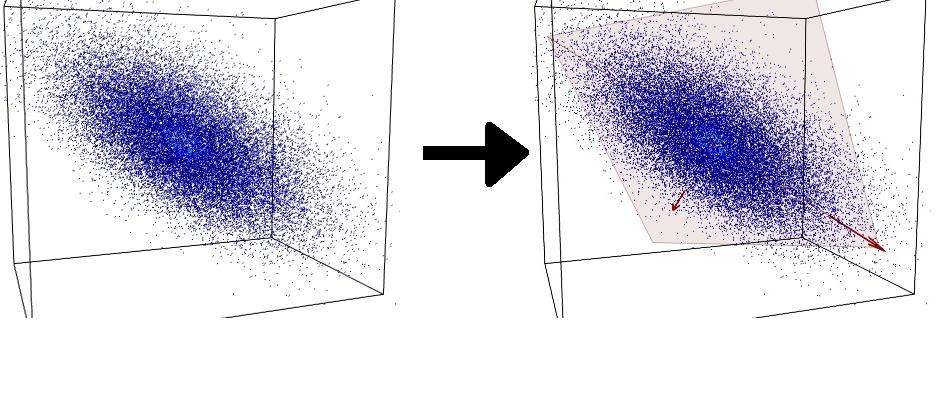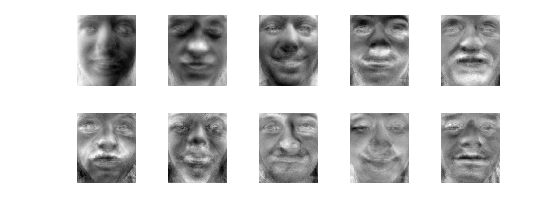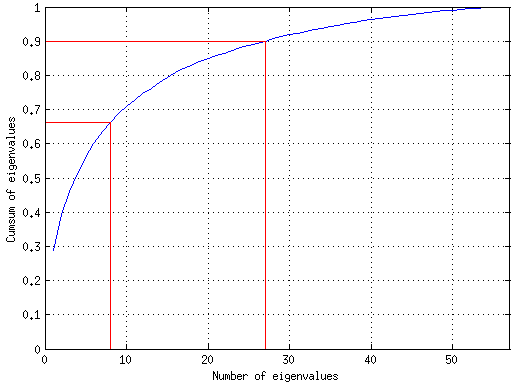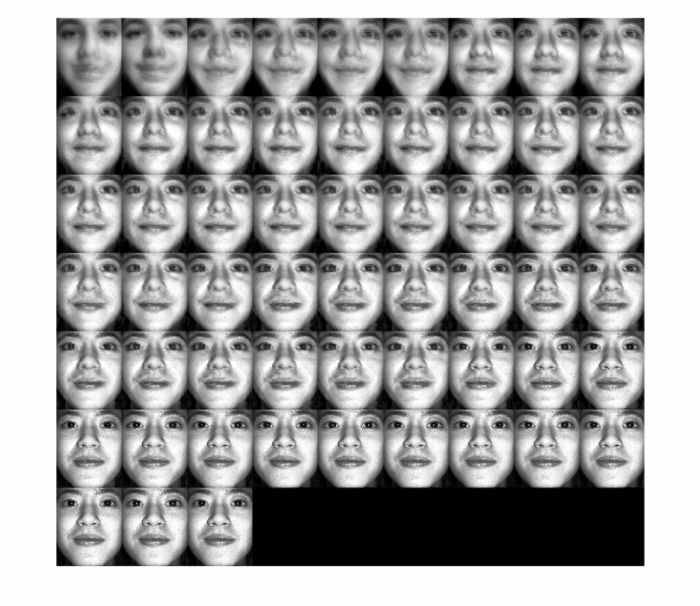Warning

# Principal Component Analysis (PCA)Figure 1. Dimensionality reduction by PCA1).

So far, in this course, we have been using measurements of various dimensionality, sometimes the measurements had even dimensionality same as the number of pixels in the image. But are all those dimensions actually necessary? Isn't there a more compact representation of the data which we could use instead without sacrificing the expressiveness of the data? Have a look at Figure 1 for an example. All the data, though 3-dimensional lay actually in a 2D plane. If we were able to compute the orthogonal basis of this plane (red arrows in Figure 1, right), we could represent the data using just 2D measurements. Principal component analysis (PCA) is a method for such features space dimensionality reduction (also called feature extraction).

In today's labs, the PCA method will be used to extract a new set of “more compact” measurements from the set of images of faces. We are given a set of images of faces of size 300×250, but in the following we will represent them rather as a column vector of intensities of length $n$ = 75000 (=300*250) - a vector of image columns stacked one after another. Thus, each image can be seen as a point in $n$-dimensional space. The goal of PCA is then to represent the images in $m$-dimensional space, where $m \ll n$.

We will then use this compact representation to classify the faces of several individuals using the nearest neighbour classifier.

## Theory

The input to PCA is a set $X=\{\mathbf{x}_i|\mathbf{x}_i \in \mathbb{R}^n, i=1,\ldots,k\}$ of $k$ training vectors, in our case $k$ vectorised images of faces. To be able to apply the PCA, the data should be centred in origin, i.e. having zero mean, $\bar{\mathbf{x}} = \frac{1}{k}\sum_{i=1}^{k} \mathbf{x}_i = \mathbf{0}$. This is usually not the case for real data, so the first step is always to centre the data by subtracting their mean: $\tilde{\mathbf{x}}_i = \mathbf{x}_i - \bar{\mathbf{x}}$. To simplify notation, we will still refer to $\mathbf{x}_i$ instead of $\tilde{\mathbf{x}}_i$ in the following and assuming that the data is already centered. However, be aware, that every time you are using the data this conversion is implicitly present and you have to take it into account in the code!

PCA searches for a set $Y = \{\mathbf{y}_j|\mathbf{y}_j \in \mathbb{R}^n, j=1,\ldots,m \}$ of $m$ vectors ($m<n$), and a set $W = \{\mathbf{w}_i|\mathbf{w}_i \in \mathbb{R}^m, i=1,\ldots,k\}$ such, that the approximated vectors (images) $$\mathbf{z}_i = \mathbf{Y}\mathbf{w}_i, \quad \mathbf{Y}= \begin{bmatrix} \mathbf{y}_1 & \cdots & \mathbf{y}_m \end{bmatrix}$$ are good approximation of the original vectors $\mathbf{x}_i$ in the least squares sense, i.e. their residuum $$\sum_{i=1}^{k} \| \mathbf{x}_i - \mathbf{z}_i \|^2$$ is minimised. Thus, each image $\mathbf{x}_i$ is approximated by a linear combination of small number of vectors $\mathbf{y}_i$. These vectors are called principal components and correspond to the red arrows in Figure 1. Note that their dimension is the same as the original vectors. In the case of images of faces they are also usually called eigenfaces. As $m < n$, each input vector $\mathbf{x}_i$ is actually represented by a smaller number of coefficients $\mathbf{w}_i$ – the dimensionality reduction was achieved!

The vectors $\mathbf{y}_j \in Y$ are found as first $m$ eigenvectors corresponding to $m$ largest eigenvalues of the matrix $\mathbf{S} = \mathbf{X}\mathbf{X}^T$, where $\mathbf{X} = \begin{bmatrix} \mathbf{x}_1 & \cdots & \mathbf{x}_k \end{bmatrix}$ is an $n \times k$ matrix containing all input images.

The coefficients $\mathbf{w}_i$ of the linear combination are then found as dot products $$\mathbf{w}_i = \mathbf{Y}^T\mathbf{x}_i\,.$$ This geometrically corresponds to a projection of $\mathbf{x}_i$ onto the orthogonal base given by the vectors $\mathbf{y}_j$.

## A useful trick

As is often the case when working with images, the matrix $\mathbf{S}$ soon becomes too large to fit in the memory. In our case, $\mathbf{S}$ would need about 45GB. Therefore, we employ the following trick , .

Recall, that for the eigenvectors and eigenvalues of $\mathbf{S}$ we have $$\mathbf{Su} = \lambda \mathbf{u}$$

Instead of $\mathbf{S}$, let us construct a smaller $k \times k$ matrix $\mathbf{T} = \mathbf{X}^T\mathbf{X}$. The relation between eigenvectors of $\mathbf{S}$ and $\mathbf{T}$ is then

$$\mathbf{Tv} = (\mathbf{X}^T\mathbf{X})\mathbf{v} = \lambda\mathbf{v}$$ $$\mathbf{X}(\mathbf{X}^T\mathbf{X})\mathbf{v} = \mathbf{X}\lambda\mathbf{v} = \lambda(\mathbf{Xv})$$ $$\mathbf{S}(\mathbf{Xv}) = \lambda(\mathbf{Xv})$$

Where $\mathbf{v}$ and $\lambda$ are the eigenvectors and eigenvalues of $\mathbf{T}$. Therefore, if we look for the first $m \leq k$ eigenvectors of $\mathbf{S}$ only, it is sufficient to compute the eigenvectors of much smaller matrix $\mathbf{T}$ and multiplying them by matrix $\mathbf{X}$ $$\mathbf{y}_j = \mathbf{X} \mathbf{v}_j\,.$$

To fulfil this assignment, you need to submit these files (all packed in a single .zip file) into the upload system:

• answers.txt - answers to the Assignment Questions
• assignment_12.m - a script for data initialization, calling of the implemented functions and plotting of their results (for your convenience, will not be checked)
• pca_basis.m - a function that computes the principal component vectors from the training data
• compact_representation.m - a function which converts the original images to the vectors of weights
• reconstruct_images.m - a function that perform linear combination in order to reconstruct the images
• classify_nn_pca.m - a function that classify the reduced representation of the images by the nearest neighbor search
• eigenfaces.png, cumulative_sum_graph.png, partial_reconstructions.png and error_vs_reduction_graph.png - images specified in the tasks

1. Implement function [Y, lambdas] = pca_basis(X) using the images from trn_data as input, i.e find the eigenvectors of the training samples (recall the trick introduced before). The input X is the matrix of vectorised images (with the mean already subtracted), the output Y is a matrix containing, as column vectors, the set of normalised eigenvectors and lambdas is the array of the corresponding normalised (sum up to one) eigenvalues for the components. Both arrays Y and lambdas must be sorted from the largest to the smallest eigenvalues.

Tip 1: Eigenvectors and eigenvalues are in MATLAB computed by function eig.

Tip 2: Normalise the computed eigenvectors to unit length, since the projection of the data points is meaningful on the orientation of the principal components.

Tip 3: eig() function returns different eigenvectors in different versions of Matlab. The difference is in their sign only, so all such solutions are valid. To unify our results, the template code enforces the first non-zero component of each eigenface vector to be positive.

2. Display the eigenvectors (eigenfaces) as images and generate the image file eigenfaces.png. Pick the first 10 eigenfaces corresponding to the greatest eigenvalues.

Tip: Reshape vectors $\mathbf{y}_j$ (columns of Y) to matrices of the same size as the input images.3. Plot the cumulative sum of normalised eigenvalues (lambdas). Use the output of the function pca_basis. Empirically, one typically selects the number of eigenvectors used to approximate the data so that the cumulative sum of their respective eigenvalues is about 65%-90% of the sum of all eigenvalues. This usually works well, however it always depends on the problem. Save the plot as an image with name cumulative_sum_graph.png4. Implement the function W = compact_representation(X, Y, m) that computes the weights of the principal components. Inputs are X, the matrix with the images of faces, Y, the matrix with the orthogonal set of principal components in column vector format, and m is the number of components to be used (the most dominant ones). The output W is a matrix with the compact representation coefficients.

5. Implement the function Z = reconstruct_images(W, Y, x_mean) with inputs W and Y are the same as above and x_mean is the vectorised mean image of the faces. The output Z is a matrix containing the approximated vectorised images as columns.

6. Using the compact_representation and reconstruct_images functions generate partial reconstructions for $m=1,2,\ldots$. Save them as a single image partial_reconstructions.png.7. Build a nearest neighbour classifier for the tst_data in the reduced-representation space (use the function compact_representation before) inside the function [labels_tst] = classify_nn_pca(images, Y_trn, X_trn_mean, W_trn, labels_trn, m). It takes the matrices obtained from the above functions plus the images (use directly tst_data.images here), and the value m of the number of components used for representation. It outputs the classification labels (integers).

Tip: Do not use the test data mean ever!

8. Experiment with the value of m (from 1 to 57) and plot the classification error versus the number of eigenvectors in a graph and save it as error_vs_reduction_graph.png. Use labeling in labels_tst_gt to evaluate the error.

## Assignment Questions

1. What would be a good indicator in the data set for applying PCA?
• a) Symmetric covariance matrix
• b) Correlation between measurements
• c) Data set was generated with respect to a given probability distribution
• d) Measurements have very different nature
2. The principal components dimensionality is:
• a) smaller than the original data dimensionality
• b) the same as the original data dimensionality
• c) larger than the original data dimensionality
3. An image as a 2D signal has a range of spatial frequencies (i.e. edges, homogeneous intensity regions, gradients, etc.), which frequency range is represented by the principal components with the highest eigenvalues?
• a) High frequencies
• b) Medium frequencies
• c) Low frequencies

What to do next? With the knowledge gained this semester, you can

• … experiment with another (better?) classifier in the reduced-representation space of faces,
• … try to align the faces first, i.e. before computing the PCA. (Use another data file, where the faces are not so tightly clipped, so you have some space to crop the images. For inspiration, see ),
• … experiment with PCA on letters, which were used in previous labs (e.g. with the data from the AdaBoost labs),
• … implement a similar dimensionality reduction technique called Independent Component Analysis (ICA),

Any of the above problems is considered a bonus task. The formulations are intentionally vague and the precise task formulation and implementation is part of the task.

 Eigenfaces for recognition talk

 Face alignment for increased PCA precision

 Turk, M. and Pentland, A. (1991). Eigenfaces for recognition. The Journal of Cognitive Neuroscience, 3(1): 71-86.